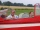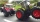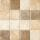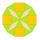# Prime numbers + area - math problems

#### Number of problems found: 24

• The landThe land, which has dimensions of 220 m and 308 m, the owner wants to divide into equally large square plots with the largest possible area. How long will one side of the plot be?
• Square gardensThe gardening colony with dimensions of 180 m and 300 m is to be completely divided into equally large square areas with the largest possible area. Calculate how many such square areas can be obtained and determine the side length of the square.
• How manyHow many different rectangles with integer page lengths have an area S = 60 cm²?
• Largest squaresHow many of the largest square sheets did the plumber cut the honeycomb from 16 dm and 96 dm?
• RectanglesHow many different rectangles can be made from 60 square tiles of 1 m square? Find the dimensions of these rectangles.
• HectaresThe tractor plows the first day of 4.5 ha, the second day 6.3 ha, and the third day of 5.4 ha. It worked whole hours a day, and its hourly performance did not change and was the highest of the possible. How many hectares did it plow in one hour (what is i
• Minimum surfaceFind the length, breadth, and height of the cuboid-shaped box with a minimum surface area, into which 50 cuboid-shaped blocks, each with length, breadth, and height equal to 4 cm, 3 cm, and 2 cm respectively, can be packed.
• Two rectanglesI cut out two rectangles with 54 cm², 90 cm². Their sides are expressed in whole centimeters. If I put these rectangles together I get a rectangle with an area of 144 cm2. What dimensions can this large rectangle have? Write all options. Explain your calc
• Glass panelA rectangular glass panel with dimensions of 72 cm and 96 cm will cut the glazier on the largest square possible. What is the length of the side of each square? How many squares does the glazier cut?
• Cuboid wallsCalculate the cuboid volume if its different walls have an area of 195cm², 135cm², and 117cm².
• PagansElena cut out same circle-shaped pagans and put them on a rectangular sheet so that the neighboring pagans were touching each other and the pagans were touching the walls of the sheet on the edges. Each pagans occupied 28.26 cm2 of the bottom of the sheet
• CubesCarol with cut bar 12 cm x 12 cm x 135 cm to the cubes. Find the sum of all the surfaces of the resulting cubes.
• TilesIt is given an area of 5m x 4m. One tile is 40 x 40 cm. How many tiles are needed in an area of 5 m x 4 m? And how many tiles need to be cut (if it not possible for the tiles to fall exactly)?
• TilesHow many tiles of 20 cm and 30 cm can build a square if we have a maximum of 100 tiles?
• Square tilesThe room has dimensions of 12 meters and 5.6 meters. Determine the number of square tiles and their largest dimension to exactly cover the floor.
• Mr. ZucchiniMr. Zucchini had a rectangular garden whose perimeter is 28 meters. The garden's content area filled just four square beds, whose dimensions in meters are expressed in whole numbers. Determine what size could have a garden. Find all the possibilities and
• CuboidThe volume of the cuboid is 245 cm3. Each cuboid edge length can be expressed by an integer greater than 1 cm. What is the surface area of the cuboid?
• Paper squaresThe paper rectangle measuring 69 cm and 46 cm should be cut into as many squares as possible. Calculate the lengths of squares and their number.
• TilesThe room has dimensions 12 m and 5.6 m. Determine the number of square tiles and their largest possible size to cover them room's floor.
• PlumberThe plumber had to cut the metal strip with dimensions 380 cm and 60 cm to the largest squares to no waste. Calculate the length of the sides of a square. How many squares cut it?

Do you have an exciting math question or word problem that you can't solve? Ask a question or post a math problem, and we can try to solve it.

We will send a solution to your e-mail address. Solved examples are also published here. Please enter the e-mail correctly and check whether you don't have a full mailbox.

Prime numbers - math problems. Area - math problems.